International
Tables for
Crystallography
Volume C
Mathematical, physical and chemical tables
Edited by E. Prince

International Tables for Crystallography (2006). Vol. C, ch. 8.1, pp. 678-680

## Section 8.1.1. Definitions

E. Princea and P. T. Boggsb

aNIST Center for Neutron Research, National Institute of Standards and Technology, Gaithersburg, MD 20899, USA, and bScientific Computing Division, National Institute of Standards and Technology, Gaithersburg, MD 20899, USA

### 8.1.1. Definitions

| top | pdf |

#### 8.1.1.1. Linear algebra

| top | pdf |

A matrix is an ordered, rectangular array of numbers, real or complex. Matrices will be denoted by upper-case, bold italic letters, A. Their individual elements will be denoted by upper-case, italic letters with subscripts. Aij denotes the element in the ith row and the jth column of A. A matrix with only one row is a row vector; a matrix with only one column is a column vector. Vectors will be denoted by lower-case, bold roman letters, and their elements will be denoted by lower-case, italic letters with single subscripts. Scalar constants will usually be denoted by lower-case, Greek letters.

A matrix with the same number of rows as columns is square. If Aij = 0 for all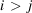, A is upper triangular. If Aij = 0 for all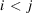, A is lower triangular. If Aij = 0 for all, A is diagonal. If Aij = 0 for all i and j, A is null. A matrix, B, such that Bij = Aji for all i and j is the transpose of A, and is denoted by AT. Matrices with the same dimensions may be added and subtracted: (A+ B)ij = Aij + Bij. A matrix may be multiplied by a scalar: (αA)ij = αAij. Multiplication of matrices is defined by (AB)ij =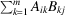, where m is the number of columns of A and the number of rows of B (which must be equal). Addition and multiplication of matrices obey the associative law: (A + B) + C = A + (B + C); (AB)C = A(BC). Multiplication of matrices obeys the distributive law: A(B + C) = AB + AC. Addition of matrices obeys the commutative law: A + B = B + A, but multiplication, except in certain (important) special cases, does not: ABBA. The transpose of a product is the product of the transposes of the factors in reverse order: (AB)T= BTAT.

The trace of a square matrix is the sum of its diagonal elements. The determinant of an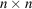square matrix, A, denoted by |A|, is the sum of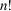terms, each of which is a product of the diagonal elements of a matrix derived from A by permuting columns or rows (see Stewart, 1973). The rank of a matrix (not necessarily square) is the dimension of the largest square submatrix that can be formed from it, by selecting rows and columns, whose determinant is not equal to zero. A matrix has full column rank if its rank is equal to its number of columns. A square matrix whose diagonal elements are equal to one and whose off-diagonal elements are equal to zero is an identity matrix, denoted by I. If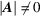, A is nonsingular, and there exists a matrix A−1, the inverse of A, such that AA−1 = A−1A = I. If |A| = 0, A is singular, and has no inverse. The adjoint, or conjugate transpose, of A is a matrix,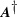, such that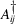=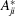, where the asterisk indicates complex conjugate. If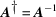, A is unitary. If the elements of a unitary matrix are real, it is orthogonal. From this definition, if A is orthogonal, it follows that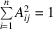for all j, and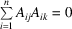if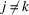. By analogy, two column vectors, x and y, are said to be orthogonal if xTy = 0.

For any square matrix, A, there exists a set of vectors,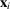, such that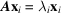, where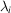is a scalar. The valuesare the eigenvalues of A, and the vectorsare the corresponding eigenvectors. If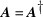, A is Hermitian, and, if the elements are real, A = AT, so that A is symmetric. It can be shown (see, for example, Stewart, 1973) that, if A is Hermitian, all eigenvalues are real, and there exists a unitary matrix, T, such that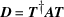is diagonal, with the elements of D equal to the eigenvalues of A, and the columns of T are the eigenvectors. An n × n symmetric matrix therefore has n mutually orthogonal eigenvectors. If the product xTAx is greater than (or equal to) zero for any non-null vector, x, A is positive (semi)definite. Because x may be, in particular, an eigenvector, all eigenvalues of a positive (semi)definite matrix are greater than (or equal to) zero. Any matrix of the form BTB is positive semi definite, and, if B has full column rank, A = BTB is positive definite. If A is positive definite, there exists an upper triangular matrix, R, or, equivalently, a lower triangular matrix, L, with positive diagonal elements, such that RTR = LLT= A. R, or L, is called the Cholesky factor of A. The magnitude, length or Euclidean norm of a vector, x, denoted by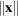, is defined by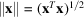. The induced matrix norm of a matrix, B, denoted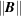, is defined as the maximum value of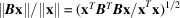for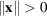. Because xTBTBx will have its maximum value for a fixed value of xTx when x is parallel to the eigenvector that corresponds to the largest eigenvalue of BTB, this definition implies that ||B|| is equal to the square root of the largest eigenvalue of BTB. The condition number of B is the square root of the ratio of the largest and smallest eigenvalues of BTB. (Other definitions of norms exist, with corresponding definitions of condition number. We shall not be concerned with any of these.)

We shall make extensive use of the so-called QR decomposition, which is defined as follows: For any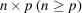real matrix, Z, there exists an n × n orthogonal matrix, Q, such that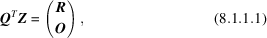where R is a p × p upper triangular matrix, and O denotes an (np) × p null matrix. Thus, we have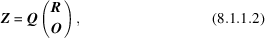which is known as the QR decomposition of Z. If we partition Q as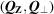, where QZ has dimensions n × p, and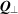has dimensions n × (np), (8.1.1.2)becomes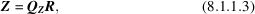which is known as the QR factorization. We shall make use of the following facts. First, R is nonsingular if and only if the columns of Z are linearly independent; second, the columns of QZ form an orthonormal basis for the range space of Z, that is, they span the same space as Z; and, third, the columns ofform an orthonormal basis for the null space of ZT, that is, ZT= O.

There are two common procedures for computing the QR factorization. The first makes use of Householder transformations, which are defined by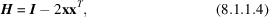where xTx = 1. H is symmetric, and H2 = I, so that H is orthogonal. In three dimensions, H corresponds to a reflection in a mirror plane perpendicular to x, because of which Stewart (1973) has suggested the alternative term elementary reflector. A vector v is transformed by Hv into the vector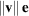, where e represents a vector with e1 = 1, and ei = 0 for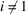, if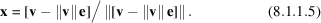The factorization procedure for an n × p matrix, A (Stewart, 1973; Anderson et al., 1992), takes as v in the first step the first column of A, and forms A1 = H1A, which has zeros in all elements of the first column below the diagonal. In the second step, v has a zero as the first element and is filled out by those elements of the second column of A1 on or below the diagonal. A2 = H2A1 then has zeros in all elements below the diagonal in the first two columns. This process is repeated (p − 2) more times, after which Q = HpH2H1, and R = Ap is upper triangular.

QR factorization by Householder transformations requires for efficiency that the entire n × p matrix be stored in memory, and requires of order np2 operations. A procedure that requires storage of only the upper triangle makes use of Givens rotations, which are 2 × 2 matrices of the form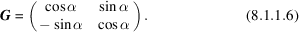Multiplication of a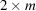matrix, B, by G will put a zero in the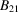element if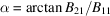. The factorization of A involves reading, or computing, the rows of A one at a time. In the first step, matrix B1 consists of the first row of R and the current row of A, from which the first element is eliminated. In the second step, B21 is the second row of R and the (p − 1) non-zero elements of the second row of the transformed B1. After the first p rows have been treated, each additional row of A requires 2p(p + 1) multiplications to fill it with zeros. However, because the operation is easily vectorized, the time required may be a small proportion of the total computing time on a vector oriented computer.

#### 8.1.1.2. Statistics

| top | pdf |

A probability density function, which will be abbreviated p.d.f., is a function, Φ(x), such that the probability of finding the random variable x in the interval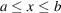is given by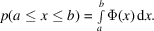A p.d.f. has the properties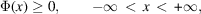and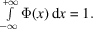A cumulative distribution function, which will be abbreviated c.d.f., is defined by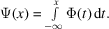The properties of Φ(x) imply that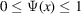, and Φ(x) = dΨ(x)/dx. The expected value of a function, f(x), of random variable x is defined by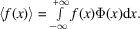If f(x) = xn,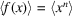is the nth moment of Φ(x). The first moment, often denoted by μ, is the mean of Φ(x). The second moment about the mean,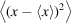, usually denoted by σ2, is the variance of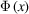. The positive square root of the variance is the standard deviation.

For a vector, x, of random variables,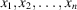, the joint probability density function, or joint p.d.f., is a function, ΦJ(x), such that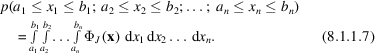The marginal p.d.f. of an element (or a subset of elements),, is a function,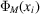, such that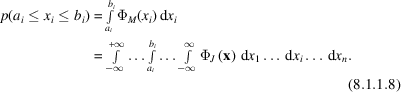This is a p.d.f. foralone, irrespective of the values that may be found for any other element of x. For two random variables, x and y (either or both of which may be vectors), the conditional p.d.f. of x given y = y0 is defined by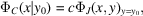where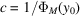is a renormalizing factor. This is a p.d.f. for x when it is known that y = y0. If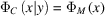for all y, or, equivalently, if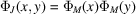, the random variables x and y are said to be statistically independent.

Moments may be defined for multivariate p.d.f.s in a manner analogous to the one-dimensional case. The mean is a vector defined by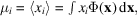where the volume of integration is the entire domain of x. The variance–covariance matrix is defined by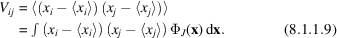The diagonal elements of V are the variances of the marginal p.d.f.s of the elements of x, that is,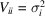. It can be shown that, ifand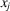are statistically independent,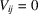when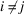. If two vectors of random variables, x and y, are related by a linear transformation, x = By, the means of their joint p.d.f.s are related by μx = Bμy, and their variance–covariance matrices are related by Vx = BVyBT.

### References

Anderson, E., Bai, Z., Bischof, C., Demmel, J., Dongarra, J., Du Croz, J., Greenbaum, A., Hammarling, S., McKenney, A., Ostrouchov, S. & Sorenson, D. (1992). LAPACK user's guide, 2nd ed. Philadelphia: SIAM Publications.
Stewart, G. W. (1973). Introduction to matrix computations. New York: Academic Press.